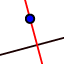# Constructing Angle Bisectors: Ex. 11

## DIRECTIONS:

1) Use the ANGLE BISECTORtool to construct the 3 angle bisectors of the triangle. 2) Use the INTERSECTtool to plot the point of concurrency (incenter) of these 3 angle bisectors. 3) Go to the algebra view (left side). Hide the 3 angle bisectors of this triangle (you've just constructed in step 1). 4) Use the PERPENDICULAR LINEtool to construct a line that passes through the incenter and is perpendicular to . Further directions appear below the applet.
5) Use the PERPENDICULAR LINE tool to construct a line that passes through the incenter and is perpendicular to . 6) Use the PERPENDICULAR LINE tool to construct a line that passes through the incenter and is perpendicular to . 7) Use the INTERSECT tool to plot the 3 points at which the lines (you constructed in steps 4 - 6) intersect the 3 sides of the triangle. 8) Go to the STEPS window. Hide the 3 lines you constructed in steps 4 - 6. 9) Construct a circle with centered at the incenter that passes through any 1 of the points you constructed in step 7.

## 10)

What do you notice? Why does this occur?

When you're done (or if you're unsure of something), feel free to check by watching the quick silent screencast below the applet.